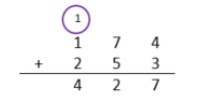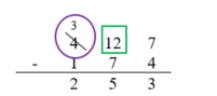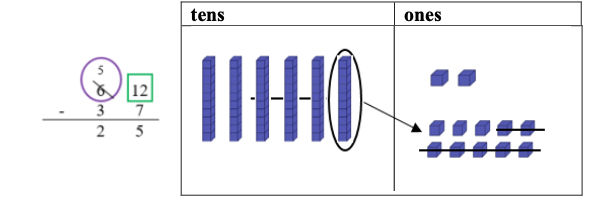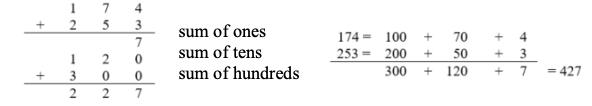# Standard #: MA.3.NSO.2.1

This document was generated on CPALMS - www.cpalms.org

Add and subtract multi-digit whole numbers including using a standard algorithm with procedural fluency.

### General Information

Subject Area: Mathematics (B.E.S.T.)
Strand: Number Sense and Operations
Status: State Board Approved

• Expression
• Equation
• Whole Number

### Vertical Alignment

Previous Benchmarks

Next Benchmarks

### Purpose and Instructional Strategies

The purpose of this benchmark is for students to add and subtract multi-digit whole numbers with procedural fluency. Students use skills from the procedural reliability stage in Grade 2 to become fluent with efficient and accurate procedures, including standard algorithms for addition and subtraction.
• A standard algorithm is defined as any efficient and accurate procedure that allows students to add and subtract whole numbers. Students’ choices of standard algorithms for addition and subtraction do not need to be the same (MTR.5.1).
• Students should be able to justify their use of a standard algorithm for adding and subtracting by explaining the steps mathematically. Each student should be able to explain if and when regrouping is needed, and how regrouping is computed using their chosen algorithm. During instruction, teachers and students should work together to relate place value understanding to algorithms (MTR.3.1, MTR.4.1, MTR.5.1).
• Problems should include both vertical and horizontal forms, including opportunities for students to apply the commutative and associative properties.
• Instruction of this benchmark should be taught with MA.3.NSO.1.4. Students should use rounding as a means to estimate reasonable solutions of sums and differences before calculating (MTR.6.1).

### Common Misconceptions or Errors

• Students who learn a standard algorithm without being able to explain why it works using place value understanding often make computational errors and/or cannot determine if their solutions are reasonable. To assist students with this misconception, teachers should expect students to justify the algorithm they choose.

### Strategies to Support Tiered Instruction

• Instruction includes guiding students through the process of estimating reasonable values for sums and differences using an understanding of place value, addition, and subtraction.
• For example, students make reasonable estimates for the sum of 174 + 253. Instruction includes a prompt such as “Before using an algorithm, we will estimate the sum to make sure that we are using the algorithm correctly and our answer is reasonable. The first addend of 174 is close to the benchmark number 200 and the second addend of 253 is close to the benchmark number 250. So, we can use 200 + 250 = 450 to estimate that our sum should be close to 450.”
• Instruction includes guiding students through the process of explaining and justifying the chosen algorithm and determining if an algorithm was used correctly by reviewing the reasonableness of solutions.
• For example, students use a standard algorithm to solve 174 + 253 and explain their thinking using a place value visual representation. Instruction includes a prompt such as “Begin by adding in the one's place. 4 ones plus 3 ones is 7 ones. Because the total number of ones is less than 10, it is not necessary to regroup. Next, add in the tens place. 7 tens plus 5 tens is 12 tens. Because I have more than 10 tens it is necessary to regroup the 10 tens to make one hundred. After composing a group of 10 tens there are 2 tens remaining. Finally, add 1 hundred plus 2 hundreds. Add the 1 hundred that was regrouped from the tens place. The sum is 427. Our sum of 427 is close to our estimate of 450, this helps us determine that our answer is reasonable”• For example, students use a standard algorithm to solve 327 − 174 and explain their thinking using a place value visual representation. Instruction includes prompts such as “ Begin subtracting 174 starting in the one's place. 7 ones minus 4 ones are 3 ones. There are not enough tens to subtract 7 tens from 2 tens. It is necessary to decompose one hundred into 10 tens. Now there are 12 tens, and there is enough to subtract 7 tens. 12 tens minus 7 tens equals 5 tens. Finally, subtract the hundreds: 3 hundreds minus 1 hundred equals 2 hundreds. The difference is 253.”• For example, students use a standard algorithm and base-ten blocks to solve 62 − 37 and explain their thinking using a place value visual representation. Instruction includes a prompt such as “Begin subtracting 37 starting in the one's place. There are not enough ones to subtract 7 ones from 2 ones. It is necessary to decompose one ten into 10 ones. Now there are 12 ones and there is enough to subtract 7 ones. 12 ones take away 7 ones equals 5 ones. Finally, subtract the tens: 5 tens minus 3 tens is 2 tens. The difference is 25.”• Teacher provides guidance on using strategies based on place value to add and subtract.
• For example, students use strategies based on place value to solve 174 + 253.• Miranda finds 492 seashells during her vacation. She now has 1,045 seashells in her collection. How many seashells did she have in her collection before vacation?
• Part A. Solve using a standard algorithm.
• Part B. Indicate one step where you needed to regroup while solving and show how you did it using words or a pictorial model.

### Instructional Items

Instructional Item 1

• What is the sum of 1,432 and 2,981?

Instructional Item 2

• What is the difference of 8,000 and 1,432?
*The strategies, tasks and items included in the B1G-M are examples and should not be considered comprehensive.

#### Related Courses

 Course Number1111 Course Title222 5012050: Grade Three Mathematics (Specifically in versions: 2014 - 2015, 2015 - 2022, 2022 and beyond (current)) 7712040: Access Mathematics Grade 3 (Specifically in versions: 2014 - 2015, 2015 - 2018, 2018 - 2022, 2022 and beyond (current)) 5012055: Grade 3 Accelerated Mathematics (Specifically in versions: 2019 - 2022, 2022 and beyond (current)) 5012015: Foundational Skills in Mathematics 3-5 (Specifically in versions: 2019 - 2022, 2022 and beyond (current))

#### Related Access Points

 Access Point Number Access Point Title MA.3.NSO.2.AP.1 Apply a strategy to add and subtract two two-digit whole numbers.

#### Educational Games

 Name Description Ice Ice Maybe: An Operations Estimation Game This fun and interactive game helps practice estimation skills, using various operations of choice, including addition, subtraction, multiplication, division, using decimals, fractions, and percents. Various levels of difficulty make this game appropriate for multiple age and ability levels. Addition/Subtraction: The addition and subtraction of whole numbers, the addition and subtraction of decimals. Multiplication/Division: The multiplication and addition of whole numbers. Percentages: Identify the percentage of a whole number. Fractions: Multiply and divide a whole number by a fraction, as well as apply properties of operations. Estimator Quiz In this activity, students are quizzed on their ability to estimate sums, products, and percentages. The student can adjust the difficulty of the problems and how close they have to be to the actual answer. This activity allows students to practice estimating addition, multiplication, or percentages of large numbers. This activity includes supplemental materials, including background information about the topics covered, a description of how to use the application, and exploration questions for use with the java applet.

#### Educational Software / Tool

 Name Description Arithmetic Quiz In this activity, students solve arithmetic problems involving whole numbers, integers, addition, subtraction, multiplication, and division. This activity allows students to track their progress in learning how to perform arithmetic on whole numbers and integers. This activity includes supplemental materials, including background information about the topics covered, a description of how to use the application, and exploration questions for use with the java applet.

#### Original Student Tutorials

 Name Description Responsibility to Vote Part 2: Graphing Data Learn how to use a bar graph to summarize voting results at school in this interactive tutorial.  This is part 2 in a two-part series. Click HERE to open Part 1. Responsibility to Vote Part 1 United States citizens have a responsibility to vote. In this integrated civics and math tutorial, a class collects voting data to display in a table showing the students' and teachers' choices for a new school project. Adding It All Up with a Standard Algorithm Learn to add multi-digit numbers using a standard algorithm in this interactive tutorial. Speedy Sam Help Speedy Sam add and subtract as quickly as possible by using the properties of addition and subtraction in this interactive tutorial.

 Name Description To regroup or not to regroup This task presents an incomplete problem and asks students to choose numbers to subtract (subtrahends) so that the resulting problem requires different types of regrouping. This way students have to recognize the pattern and not just follow a memorized algorithm--in other words, they have to think about what happens in the subtraction process when we regroup. This task is appropriate to use after students have learned the standard US algorithm.

#### Tutorials

 Name Description Subtracting: regrouping twice In this video tutorial from Khan Academy, learn how to subtract in situations that require regrouping twice using the expanded forms of numbers, as well as the standard algorithm. Mental technique for subtraction without regrouping In this Khan Academy video tutorial, consider an alternate algorithm for subtracting multi-digit numbers mentally. This video is best for students that are already comfortable with using regrouping to subtract using the standard algorithm.

#### Original Student Tutorials

 Name Description Responsibility to Vote Part 2: Graphing Data: Learn how to use a bar graph to summarize voting results at school in this interactive tutorial.  This is part 2 in a two-part series. Click HERE to open Part 1. Responsibility to Vote Part 1: United States citizens have a responsibility to vote. In this integrated civics and math tutorial, a class collects voting data to display in a table showing the students' and teachers' choices for a new school project. Adding It All Up with a Standard Algorithm: Learn to add multi-digit numbers using a standard algorithm in this interactive tutorial. Speedy Sam: Help Speedy Sam add and subtract as quickly as possible by using the properties of addition and subtraction in this interactive tutorial.

#### Educational Games

 Name Description Ice Ice Maybe: An Operations Estimation Game: This fun and interactive game helps practice estimation skills, using various operations of choice, including addition, subtraction, multiplication, division, using decimals, fractions, and percents. Various levels of difficulty make this game appropriate for multiple age and ability levels. Addition/Subtraction: The addition and subtraction of whole numbers, the addition and subtraction of decimals. Multiplication/Division: The multiplication and addition of whole numbers. Percentages: Identify the percentage of a whole number. Fractions: Multiply and divide a whole number by a fraction, as well as apply properties of operations. Estimator Quiz: In this activity, students are quizzed on their ability to estimate sums, products, and percentages. The student can adjust the difficulty of the problems and how close they have to be to the actual answer. This activity allows students to practice estimating addition, multiplication, or percentages of large numbers. This activity includes supplemental materials, including background information about the topics covered, a description of how to use the application, and exploration questions for use with the java applet.

#### Educational Software / Tool

 Name Description Arithmetic Quiz: In this activity, students solve arithmetic problems involving whole numbers, integers, addition, subtraction, multiplication, and division. This activity allows students to track their progress in learning how to perform arithmetic on whole numbers and integers. This activity includes supplemental materials, including background information about the topics covered, a description of how to use the application, and exploration questions for use with the java applet.

 Name Description To regroup or not to regroup: This task presents an incomplete problem and asks students to choose numbers to subtract (subtrahends) so that the resulting problem requires different types of regrouping. This way students have to recognize the pattern and not just follow a memorized algorithm--in other words, they have to think about what happens in the subtraction process when we regroup. This task is appropriate to use after students have learned the standard US algorithm.

#### Tutorials

 Name Description Subtracting: regrouping twice: In this video tutorial from Khan Academy, learn how to subtract in situations that require regrouping twice using the expanded forms of numbers, as well as the standard algorithm. Mental technique for subtraction without regrouping: In this Khan Academy video tutorial, consider an alternate algorithm for subtracting multi-digit numbers mentally. This video is best for students that are already comfortable with using regrouping to subtract using the standard algorithm.